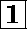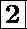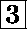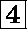### Introduction to ComputersCS-101(B1) / Fall 1997Give the truth table for the 3-input ``equivalence'' circuit (also known as the ``unanimous'' circuit), which is defined as follows.. It accepts three inputs W, X, and Y and produces one output Z. The output of the circuit should be 1 only when W, X, and Y are the same (i.e. all three are 0 or all three are 1).Using the truth table you obtained in the previous question, construct a logical circuit (using only AND, OR, and NOT gates) that implements the 3-input ``equivalence'' circuit.Let A and B be two bytes, where A = 01101011 and B = 00111011. What is the decimal value of A? What is the decimal value of B? Perform an addition of A and B in binary showing the sum and carry for each bit. What is the decimal value of the sum? Does it check with the sum of the decimal values of A and B?Give the representation of the decimal number 176 in binary (radix = 2), in ternary (radix = 3), and in octal (radix = 8). How many digits would you need to represent the number 1234 in hexadecimal (radix = 16)?Show the truth table for the ``sum'' function and the ``carry'' function for the full adder described in class. Draw a diagram for a circuit to add 3-bit numbers using 3 full adders.

```Created on: 1997.11.13
Updated on: 1997.11.13
Maintainer: Azer Bestavros
best@cs.bu.edu
```